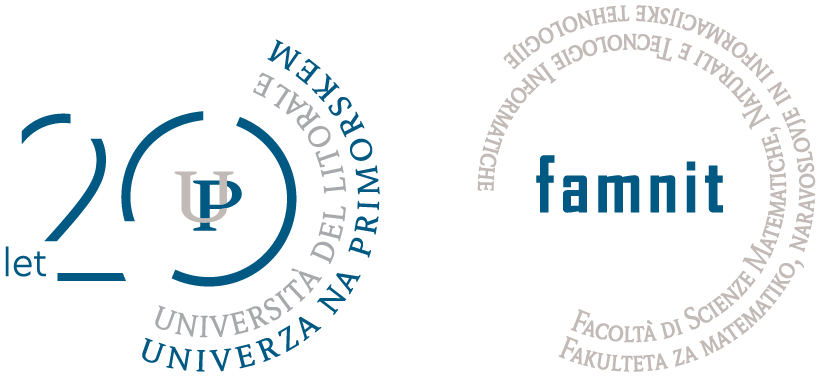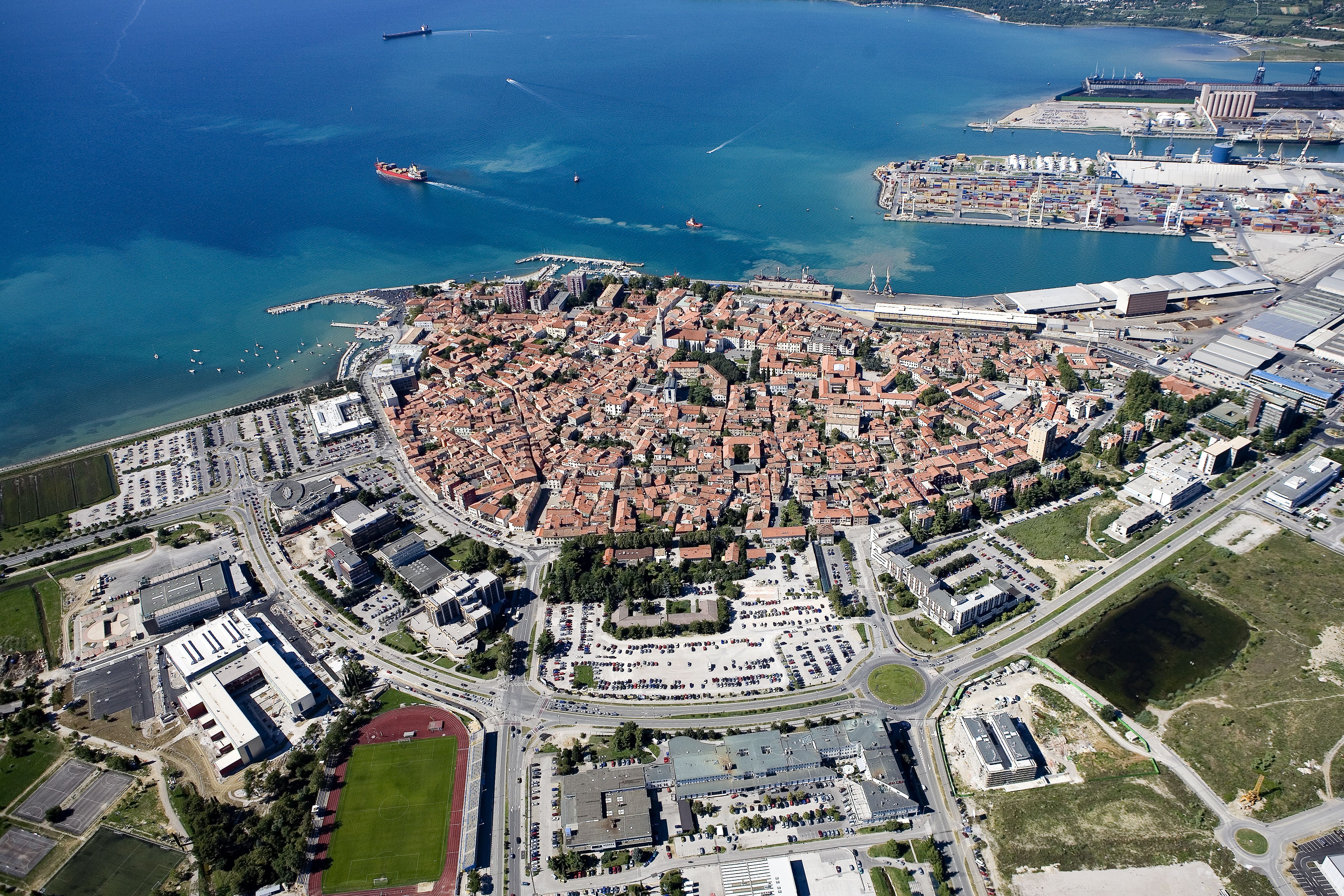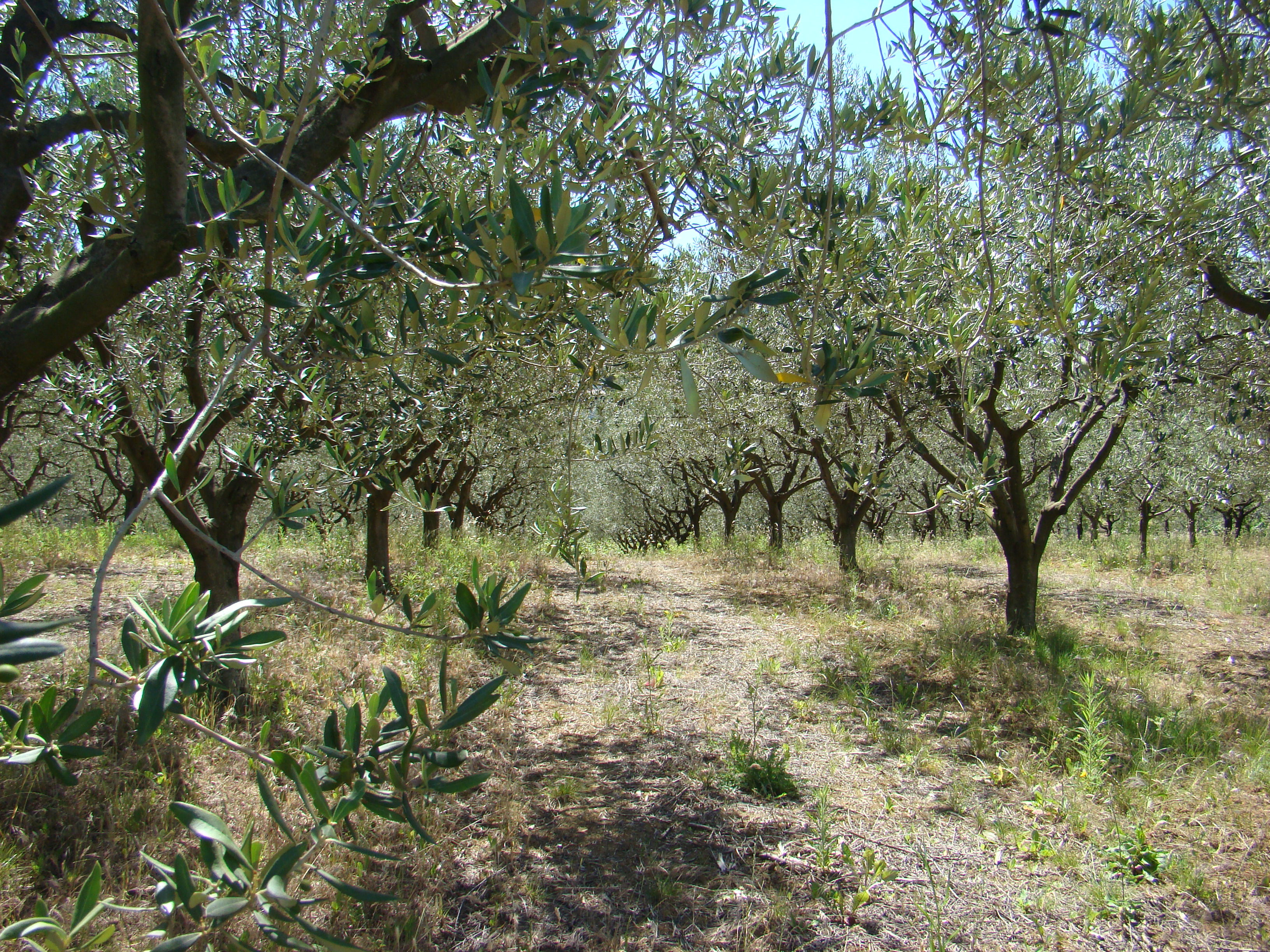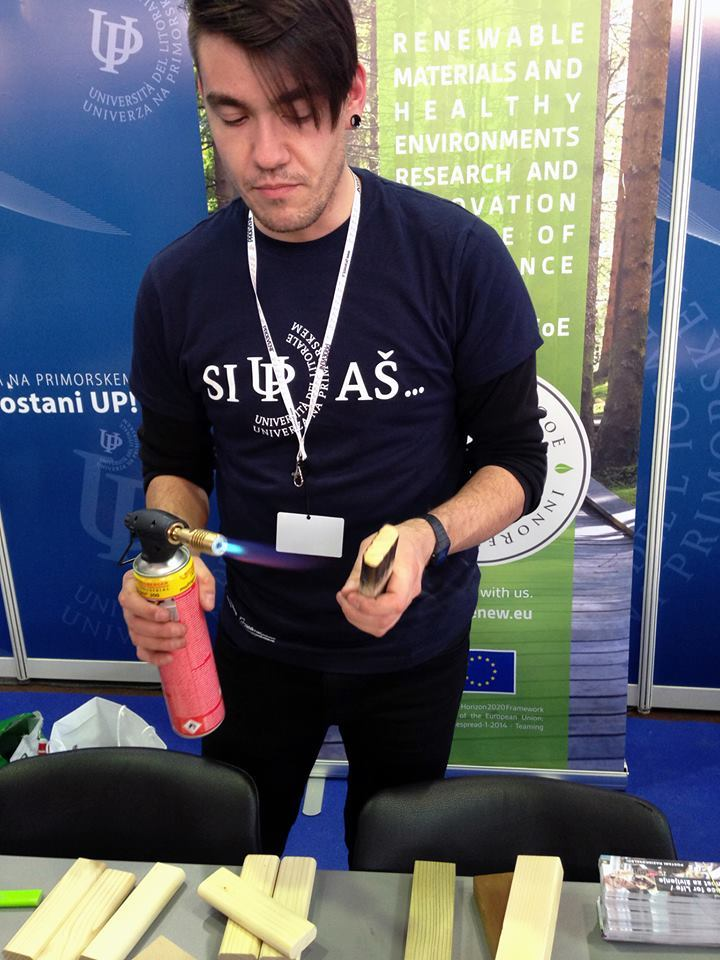Univerza na Primorskem Fakulteta za matematiko, naravoslovje in informacijske tehnologijeSI | EN# Raziskovalni matematični seminar - Arhiv

 2023 2022 2021 2020 2019 2018 2017 2016 2015 2014 2013 2012 2011 2010
 1 2 3 4 5 6 7 8 9 10 11 12

30.01.2012 Lecturer: Alexander Vasilyev

Title: Introduction to Sage. Examples of application to Graph theory

Abstract: Sage (previously SAGE) is mathematical software with features covering many aspects of mathematics, including algebra, combinatorics, numerical mathematics, number theory, and calculus. In this talk we present Sage and provide some examples for usage in Graph theory. Moreover, we will talk about the stucture of the math chemistry package we are working on.

23.01.2012 Lecturer: Samed Bajrić

Title: Almost Perfect Nonlinear functions

Abstract: In this talk we present some basic properties of Almost Perfect Nonlinear (APN) functions over finite field of characteristic 2 and investigate some open problems. We provide the characterizations of Almost Perfect Nonlinear functions and of APN permutations by means of their component functions, which can be used in an iterated secret-key block cipher as a round function to protect it from a differential cryptanalysis. We conclude with the list of all, up to equivalence, APN and Almost Bent (AB) functions, which are equivalent to certain power functions f: GF(2^n) --> GF(2^n), f(x) = x^k.

16.01.2012 Lecturer: dr. Gyorgy Kiss (Eötvös Loránd University, Budapest).

Title: Semiarcs and semiovals in PG(2, q)

Abstract : Ovals, k-arcs and semiovals of ﬁnite projective planes are not only interesting geometric structures, but they have important applications to coding theory and cryptography, too. Semiarcs are the natural generalizations of arcs. Let Πq be a projective plane of order q. A non-empty pointset St ⊂ Πq is called a t-semiarc if for every point P ∈ St there exist exactly t lines  1 , 2 , . . . t such that St ∩ i = {P } for i = 1, 2, . . . , t. These lines are called the tangents to St at P. The classical examples of semiarcs are the semiovals (t = 1) and the subplanes (t = q − m, where m is the order of the subplane.)      In this talk we consider those semiarcs in PG(2, q), which are contained in the union of three concurrent lines. Applying theorems coming from additive group theory, we prove bounds on the sizes of these semiarcs and construct new examples. We investigate strong semiovals, too. For p prime, the complete classiﬁcation of strong semiovals in PG(2, p2 ), and the proof of the nonexistence of these objects in PG(2, p) was given in [?]. We extend these results for arbitrary powers of p. In particular we prove that there is no strong semioval in PG(2, p2r+1 ) if p > 7, and prove that the size of each strong semioval in PG(2, p2r ) is 3(p2r − pr ).

09.01.2012 Lecturer: dr. Gabriel Verret

Title: Constructing a census of small cubic vertex-transitive graphs.

Abstract: We describe some recent theoretical results and various tricks which allowed us to compute a census of all cubic vertex-transitive graphs of order at most 1280. We also discuss how these methods could be expanded to higher order or valency. This is joint work with Primoz Potocnik and Pablo Spiga.